# DataWeave filterObject function: How to filter key/value pairs in an Object

We would like to thank MuleSoft Ambassador Joshua Erney for their contribution to this developer tutorial.

The `filterObject` function is similar to the `filter` function, but instead of removing items from Arrays, the `filterObject` function removes `key:value` pairs from Objects. In this tutorial, you’ll learn how to write this function in different notations, as well as using the dollar-sign syntax.

You can try all of these examples with the DataWeave Playground. To learn more about it, check out this tutorial.

## Prerequisites

While not required to follow this tutorial, a good understanding of the basic DataWeave concepts would be preferred. You can check out these other tutorials if you feel a bit lost with some concepts:

## Syntax

`filterObject` takes in an Object and a lambda that returns a Boolean. It then returns an Object with the same types as the input Object. The key difference to be aware of as a developer is that the lambda takes three parameters instead of two. It takes the value (`V`), key (`K`), and index (`Number`) of the current iteration, so you can filter based on any of those parameters.

No matter what type is used to create Object keys, they are always coerced to type Key. Even if the lambda returned a Number, the keys of the output Object would ultimately be of type Key.

## Prefix Notation

This function is not commonly used with the prefix notation because of its complexity. But here you can find an example to filter an input Object to only include the key/value pairs in which the value is a String.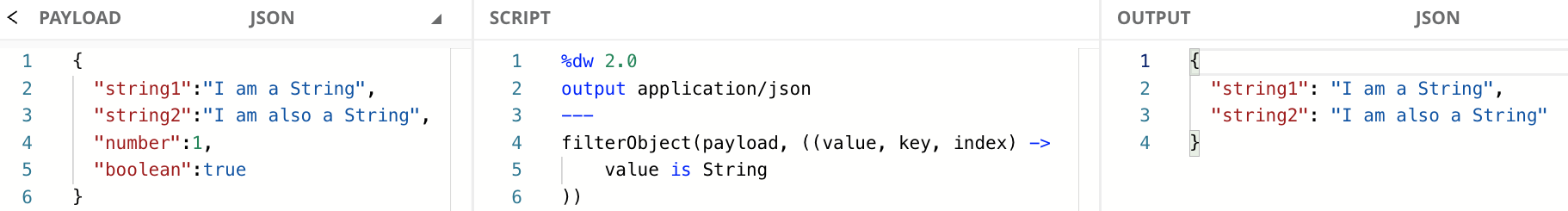## Infix Notation

This is the most used notation for this function because it makes it easier to read and understand.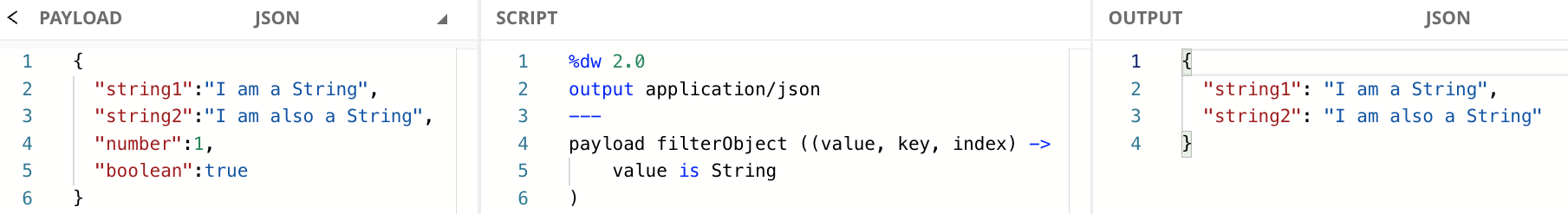When using the infix notation, you don’t have to include all the parameters in the lambda if you’re not using them. However, you do have to include the previous parameters (to the left) whether you’re using them or not. You can only remove the parameters to the right.

In this case, since `value` is the first parameter, we can also use the following code:

## Dollar-sign Syntax

You don’t always need to include the whole explicit lambda expression. You can use the dollar-sign syntax to reference the three arguments that are passed to the lambda (i.e., `value`, `key`, and `index`). `\$` represents the first argument: the value from the key/value pair (`value`), `\$\$` represents the second argument: the key from the key/value pair (`key`), and `\$\$\$` represents the third argument: the index for each key/value pair (`index`).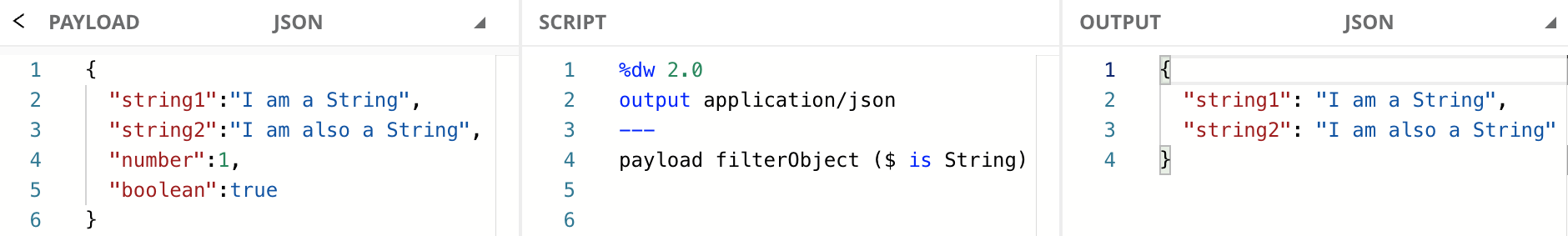## Filter Key/Value Pairs by Value

We just saw some examples to filter the key/value pairs based on the value. Let’s see a different example. Now we are filtering all the `null` values from the input Object.Note how the `postalCode` field was not removed from the final output. This is because the `filterObject` function only takes into account the first level of key/value pairs it finds. In this case, the key `address` doesn’t have a `null` value. It in fact contains an Object with more key/value pairs.

When using the infix notation, you don’t have to include all the parameters in the lambda if you’re not using them. In this case, since `value` is the first parameter we can also use the following code:

Finally, here is how the previous code would look like with the dollar-sign syntax:

If you wanted to be able to read all the “leaf values” from the Object, check out the `mapLeafValues` function from the `Tree` module in the documentation.

## Filter Key/Value Pairs by Key

Now let’s say that you wanted to include only the `name` field in the output Object. All Object keys in DataWeave are of type Key, regardless of how the Object keys are created. Because of this, we need to use the “similar to” operator instead of the “equal to” operator.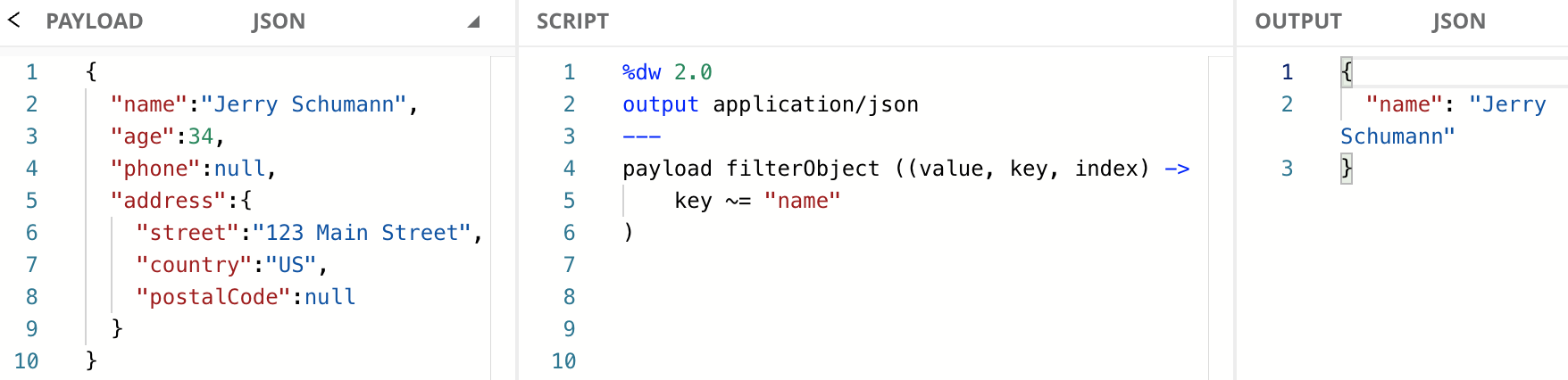To learn more about comparing different data types with the equality operators, check out this developer tutorial.

When using the infix notation, you don’t have to include all the parameters in the lambda if you’re not using them. In this case, since `key` is the second parameter we can also use the following code:

Note that we do have to include the `value` parameter. If we didn’t include the previous parameter, DataWeave would assume this was our first parameter, which is the value. Finally, here is how the previous code would look like with the dollar-sign syntax:

## Filter Key/Value Pairs by Index

Filtering by index might seem odd for Objects because the order of `key:value` pairs is not normally significant, but in DataWeave it is. When indexing Objects for these functions, DataWeave starts at the “top” of the Object and works its way to the “bottom.” In the following example, we are filtering all the key/value pairs which index is less than `3`.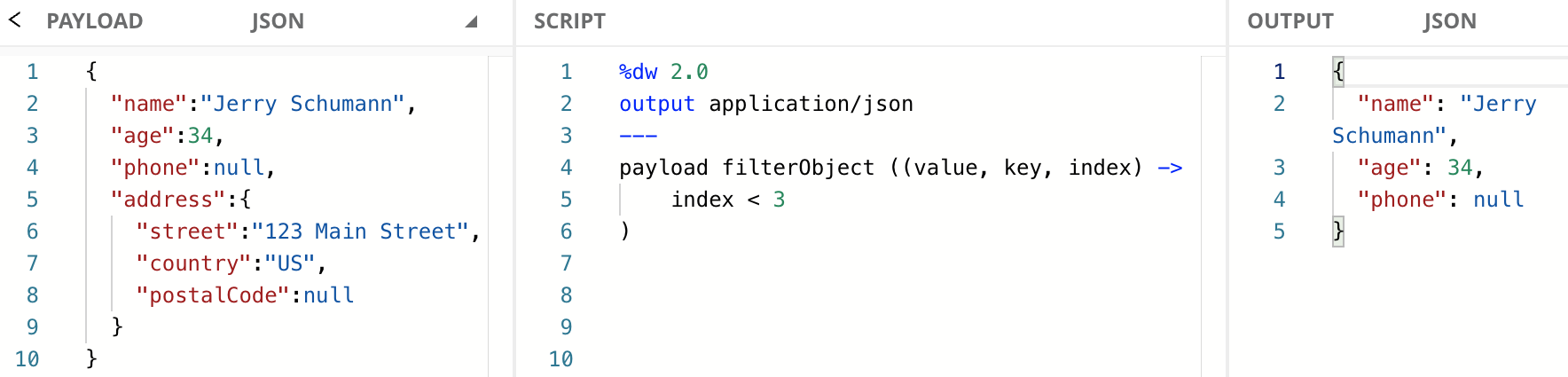When using the infix notation, you don’t have to include all the parameters in the lambda if you’re not using them. However, in this case, we’re using the 3rd parameter. This means we need to include the previous parameters as well. If we just include one parameter, DataWeave will assume you’re referring to the value instead of the index. We can’t get around removing parameters from the explicit lambda for this case.

Finally, here is how the previous code would look like with the dollar-sign syntax:

## Next Steps

The `filterObject` function is similar to the `filter` function, but instead of removing items from Arrays, the `filterObject` function removes `key:value` pairs from Objects. We learned how to write this function in different notations, as well as using the dollar-sign syntax to filter by value, key, or index.

Continue your development journey with the rest of the tutorials to become a master in DataWeave.